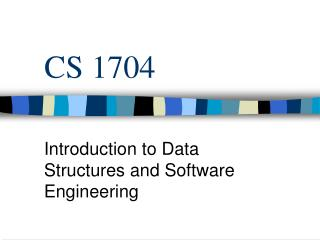DownloadDownload PresentationCS 1704

# CS 1704

Télécharger la présentation## CS 1704

- - - - - - - - - - - - - - - - - - - - - - - - - - - E N D - - - - - - - - - - - - - - - - - - - - - - - - - - -
##### Presentation Transcript

1. CS 1704 Introduction to Data Structures and Software Engineering

2. Recursion • A process in which the result of each repetition is dependent upon the result of the next repetition

3. It Works Like Iteration • Consider the following function, which computes • N! = 1 * 2 * ... * N int Factorial(int n) { int Product = 1, Scan = 2; while ( Scan <= n ) { Product = Product * Scan ; Scan = Scan + 1 ; } return (Product) ; }

4. Recursive Version int Factorial(int n ) { if ( n > 1 ) return( n * Factorial (n-1) ); else return(1); }

5. Recursion • Every recursive algorithm can be implemented non-recursively. • recursion <==> iteration • Eventually, the routine must not call itself, allowing the code to "back out". • Recursive routines that call themselves continuously are termed: • infinite recursion <==> infinite loop • Problem with recursive factorial implementation? Negative numbers! • Recursion is inefficient at runtime.

6. Array Summation // X[] array of integers to be summed // Start start summing at this index . . . // Stop . . . and stop summing at this index // int SumArray(constint X[], int Start, int Stop) { // error check if (Start > Stop || Start < 0 || Stop < 0) return 0; elseif (Start == Stop) // base case return X[Stop]; else// recursion return (X[Start] + SumArray(X, Start + 1, Stop)); }

7. // return values: SumArray(X, 0, 4) // == 134 return(X+SumArray(X,1,4)) // == 37 + 97 return(X+SumArray(X,2,4)) // == 14 + 83 return(X+SumArray(X,3,4) ) // == 22 + 61 return(X+SumArray(X,4,4)) // == 42 + 19 return X // == 19 The call:constint Size = 5;int X[Size] = {37, 14, 22, 42, 19};SumArray(X,0,Size- 1);// note Stop is last valid index

8. Coding Recursion • Solve the trivial "base" case(s). • Restate general case in 'simpler' or 'smaller' terms of itself. • Divide and Conquer • List Example • Determine the size of a single linked list. • Base Case : Empty List, size = 0 • General Case : 1 + Size(Rest of List)

9. Linked List Recursion int LinkList::SizeList () { return(listSize(Head)); } //private function: listSize int LinkList::listSize (LinkNode* list) { if (list == NULL) return( 0 ); else return( 1 + listSize(list->getNext()) ); }

10. Trace of SizeList Trace listSize(list) • listSize(list=(6, 28, 120, 496))= (1 + listSize(list=(28, 120, 496)))= (1 + (1 + listSize(list=(120, 496))))= (1 + (1 + (1 + listSize(list=(496)))))= (1 + (1 + (1 + (1 + listSize(list=(•))))))= (1 + (1 + (1 + (1 +0))))= (1 + (1 + (1 + 1)))= (1 + (1 + 2))= (1 + 3) = 4

11. Types of Recursion • Tail recursive • functions are characterized by the recursive call being the last statement in the function, (can easily be replaced by a loop). • Head Recursive • Recursive call is the first statement (maybe after a base case check) • Middle Decomposition

12. void intComma ( long num ) {if (num < 0) {// display sign for negativescout << '-'; num = -num; }if (num < 1000)cout << setw(3) << num;else { intComma(num / 1000); //Head recursivecout << ','; // display digits num = num % 1000; // separately cout << (num / 100); // for zeroes num = num % 100; cout << (num / 10) << (num % 10); }}

13. Middle Decomposition int rMax(constint ray[], int start, int end ) { constint Unknown = -1; int mid, h1max, h2max; if (end < start) return Unknown; mid = (start + end) / 2; h1max = rMax(ray, start, mid-1);//left half if (h1max == Unknown) h1max = start; h2max = rMax(ray, mid+1, end); //right half if (h2max == Unknown) h2max = end; if ( (ray[mid] >= ray[h1max]) && (ray[mid] >= ray[h2max]) ) return mid; else return( (ray[h1max]>ray[h2max]) ? h1max : h2max); }

14.      56 23 66 44 78 ray = rMax Trace • Give trace of the following array: • Give start, end, and return for each call, including the base cases in the order they RETURNED